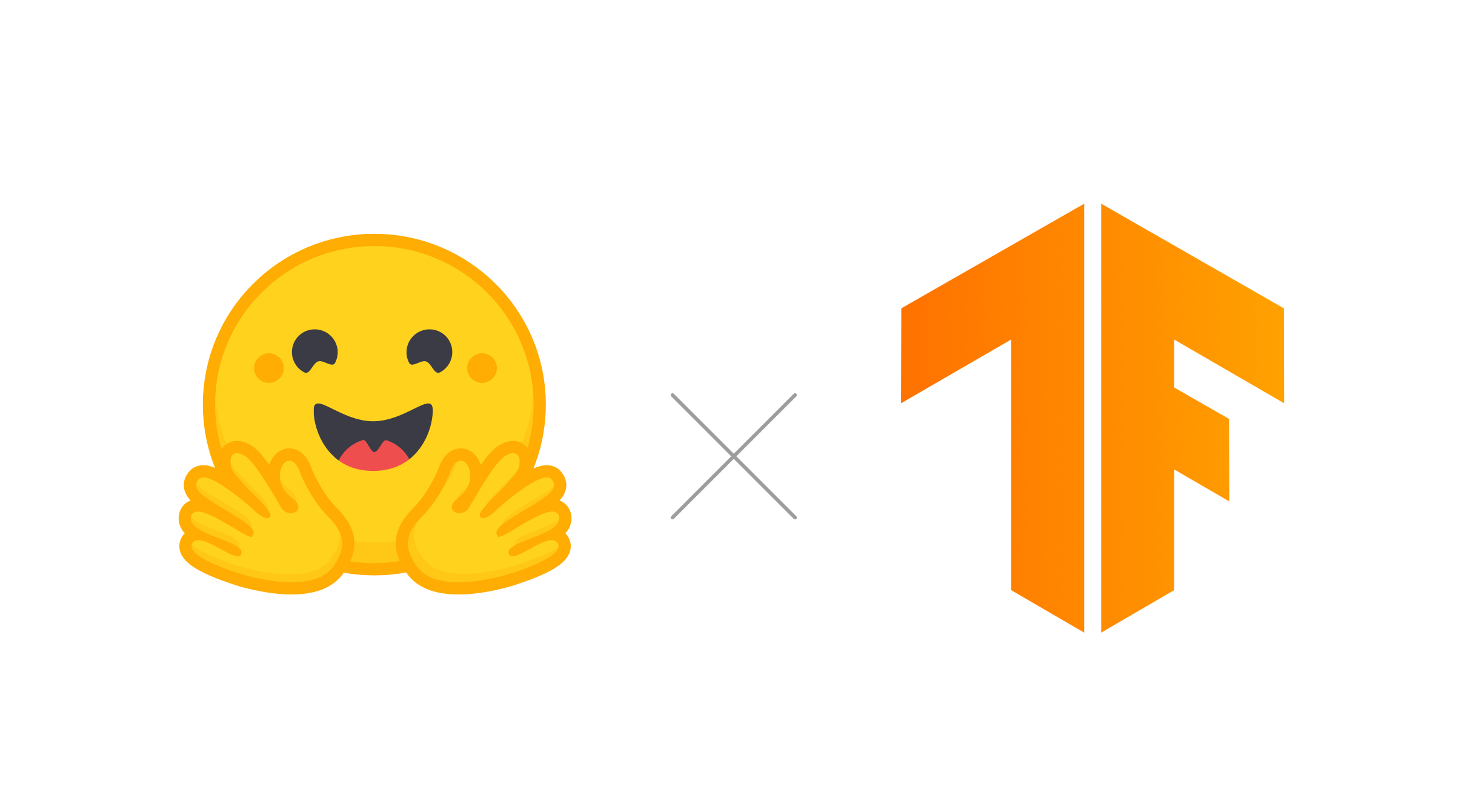# 如何基于 TensorFlow 2.0 用十行代码实现性能最佳的 NLP 模型？TensorFlow 在自然语言处理中占有重要的一席之地。Transformer 是由 Google 在 Attention Is All You Need 这篇论文中提出，其后可谓红遍大江南北，到目前为止仿佛有种“此生不识 Transformer，就称英雄也枉然”的感觉。本文讲述了如何基于 TensorFlow 2.0 实现最佳性能的自然语言处理模型，而且仅仅只需十行代码即可实现。

Hugging Face 是领先的自然语言处理初创公司，包括 Bing、Apple、Monzo 在内，有一千多家公司在生产环境中使用他们的库。本文中使用的所有示例，都可以在 Colab 上找到。这些链接可以在相应部分中找到。

## 介绍

Hugging Face 是一家专注于自然语言处理的初创公司，它拥有一个大型开源社区，尤其是 Transformers 库相关。🤗 Transformer 是一个基于 Python 的库，它公开了 API 来使用许多知名的 Transformer 架构，例如 BERTRoBERTaGPT-2DistilBERT 等，这些架构在各种自然语言处理任务（如文本分类、信息提取、问题回答和文本生成等）上获得最先进的结果。这些架构都已经经过几组权重的预训练。要开始使用 Transformer，只需安装 Pip 包：

pip install transformers

## 库及其哲学

Transformer 是基于预训练的 Transformer 模型的概念。这些 Transformer 模型具有不同的形状、大小和架构，并且有自己接收输入数据的方式：通过标记化（tokenization）。

• 配置类： 配置类包含于我们将要使用的模型相关的信息，例如层的数量和注意力头（attention head）的数量。下面是 BERT 配置文件的一个示例代码，用于预训练的权重 pre-base-cased。配置类通过各种 I/O 方法和标准化名称属性来托管这些属性。

{  "attention_probs_dropout_prob": 0.1,  "hidden_act": "gelu",  "hidden_dropout_prob": 0.1,  "hidden_size": 768,  "initializer_range": 0.02,  "intermediate_size": 3072,  "max_position_embeddings": 512,  "num_attention_heads": 12,  "num_hidden_layers": 12,  "type_vocab_size": 2,  "vocab_size": 28996}

bert-base-cased 配置文件作为 JSON。

• 词法分析类： 词法分析（Tokenzer）类负责将 Python 字符串或整数张量，这些是模型词汇表中的索引。它有许多方便的特性，可以将字符串标记化为标记。这种标记化因模型而异，因此每个模型都有自己的词法分析器。

AI 前线注： 词法分析（英语：tokenizer，lexical analysis）是计算机科学中将字符串行转换为标记（token）串行的过程。进行词法分析的进程或者函数叫作词法分析器（lexical analyzer，简称 lexer），也叫扫描器（scanner）。词法分析器一般以函数的形式存在，供语法分析器调用。

• 模型类： 模型类拥有神经网络建模逻辑本身。当使用 TensorFlow 模型时，它继承了 tf.keras.layers.Layer，这意味着它可以非常简单地使用 Keras 的 fit API，或者使用自定义的训练循环和 GradientTape 对其进行训练。

## 快乐而简单

from transformers import (TFBertModel, BertTokenizer,                         TFGPT2Model, GPT2Tokenizer) bert_model = TFBertModel.from_pretrained("bert-base-cased")  # Automatically loads the configbert_tokenizer = BertTokenizer.from_pretrained("bert-base-cased") gpt2_model = TFGPT2Model.from_pretrained("gpt2")  # Automatically loads the configgpt2_tokenizer = GPT2Tokenizer.from_pretrained("gpt2")

## 微调 Transformer 模型

• 加载文本数据并对其进行预处理。
• 定义超参数。
• 训练（使用 CPU/GPU 上的 Keras 和 TPUStrategy）

### 构建输入管道

import tensorflow_datasetsfrom transformers import glue_convert_examples_to_features data = tensorflow_datasets.load("glue/mrpc") train_dataset = data["train"]validation_dataset = data["validation"] train_dataset = glue_convert_examples_to_features(train_dataset, bert_tokenizer, 128, 'mrpc')validation_dataset = glue_convert_examples_to_features(validation_dataset, bert_tokenizer, 128, 'mrpc')train_dataset = train_dataset.shuffle(100).batch(32).repeat(2)validation_dataset = validation_dataset.batch(64)

### 使用 Keras 的 fit 方法进行训练

optimizer = tf.keras.optimizers.Adam(learning_rate=3e-5, epsilon=1e-08, clipnorm=1.0)loss = tf.keras.losses.SparseCategoricalCrossentropy(from_logits=True)metric = tf.keras.metrics.SparseCategoricalAccuracy('accuracy')bert_model.compile(optimizer=optimizer, loss=loss, metrics=[metric]) bert_history = bert_model.fit(	bert_train_dataset, 	epochs=2, 	steps_per_epoch=115, 	validation_data=bert_validation_dataset, 	validation_steps=7)

## Transformer 现在可以访问 TensorFlow API 了，那又如何？

Transformer 库的主要卖点是它的模型无关性及其简单的 API。作为在自然语言处理中获得最先进结果的模型的前端，根据当前任务在模型之间进行切换是非常容易的。

model = TFBertForSequenceClassification.from_pretrained("bert-base-cased")tokenizer = BertTokenizer.from_pretrained("bert-base-cased") data = tensorflow_datasets.load("glue/mrpc")train_dataset = data["train"]train_dataset = glue_convert_examples_to_features(train_dataset, tokenizer, 128, 'mrpc') optimizer = tf.keras.optimizers.Adam(learning_rate=3e-5, epsilon=1e-08, clipnorm=1.0)loss = tf.keras.losses.SparseCategoricalCrossentropy(from_logits=True)metric = tf.keras.metrics.SparseCategoricalAccuracy('accuracy') model.compile(optimizer=optimizer, loss=loss, metrics=[metric])model.fit(train_dataset, epochs=3)

model = TFDistilBertForSequenceClassification.from_pretrained("distilbert-base-uncased")tokenizer = DistilbertTokenizer.from_pretrained("distilbert-base-uncased")

Lysandre Debut，机器学习工程师，供职于 Hugging Face，对自然语言处理领域充满热情。

https://medium.com/tensorflow/using-tensorflow-2-for-state-of-the-art-natural-language-processing-102445cda54a